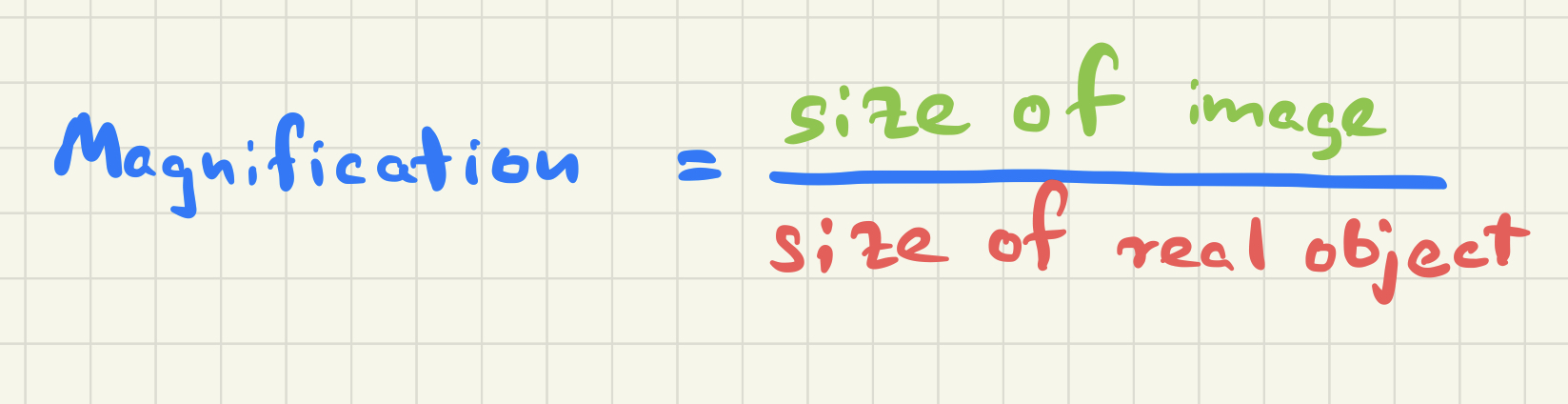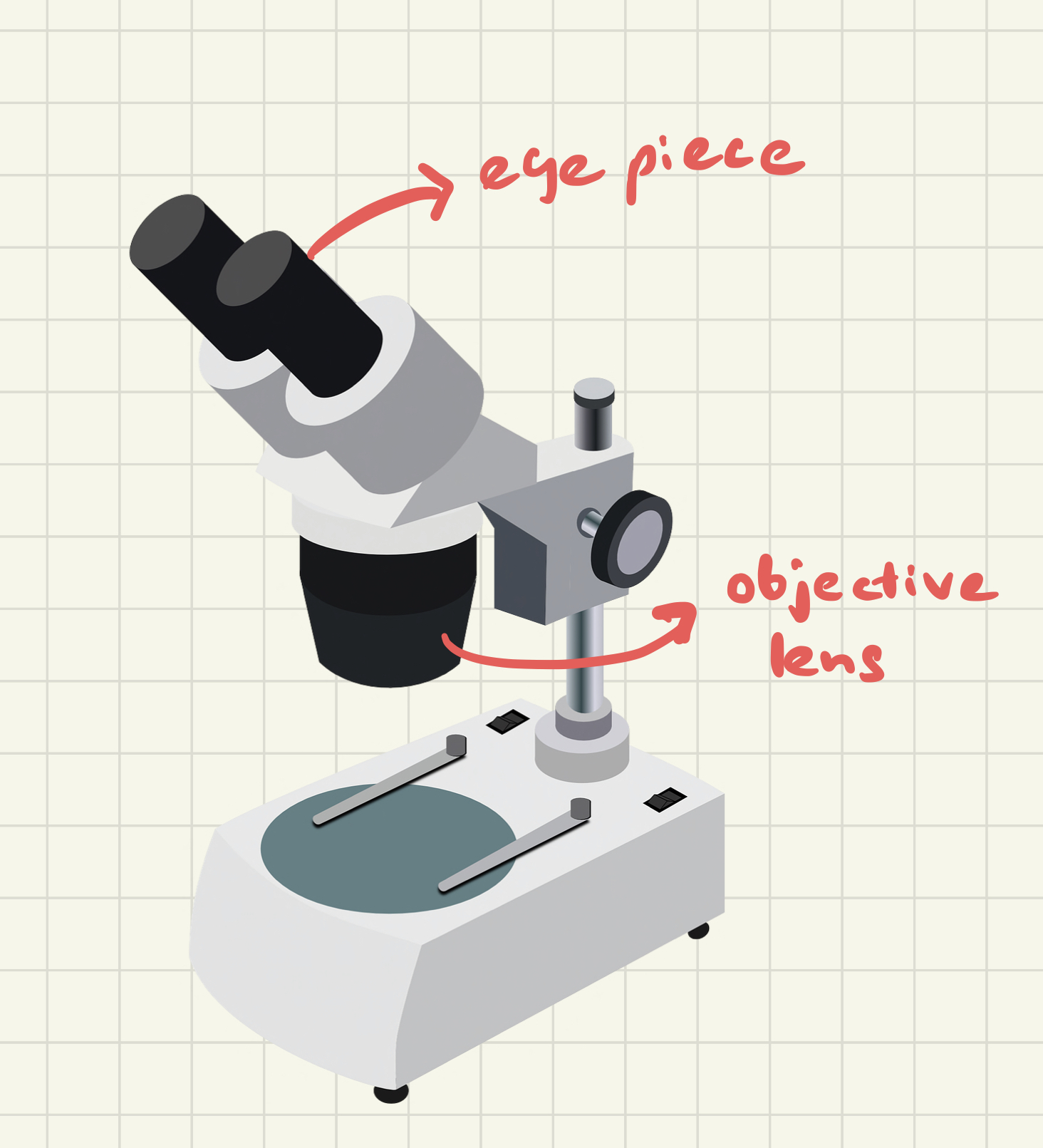Using mathematics is essential when studying biology. Let’s see this bite size math problems in cell biology lesson to understand cell biology for GCSE better. Grab a pen and a paper and let’s solve this with me.

## GCSE – Cell Biology

1. A cell 6 μm wide was magnified 2000 times. Calculate the size of the image.
2. A cell that is 8 μm in diameter appeared to be 1.2 cm in diameter when observed by a microscope. Calculate the magnification.
3. A bacterial colony of 20,000 bacteria divides every 6 minutes. How many bacteria will there be after 1 hour?
4. Calculate the cross-sectional area of a bacterial colony that is 1.2 mm in diameter.
5. The magnification of the eye piece was x10 and the magnification of the objective lens was x 40 in a particular microscopic observation. What is the magnification of the microscope?
6. Assume that an animal cell is 2.5 mm in width as seen through the microscope, at a magnification of x 400. Calculate the actual size of the cell.

## Calculating size of the image of a microscope

size of the image = magnification x real size of the real object

= 2000 x 6 μm

= 12000 μm

## Calculating the magnification power of the microscope compared to the object and the image## Calculating the size of a bacterial colony after it has multiplied

1 hour = 60 minutes

Divisions = 60 / 6

= 10

Number of bacteria after 1 hour = 20,000 x 2 10

= 2 x 104 x 1024

= 2048 x 104

cross-sectional area = πr2

= 3.142 x 1.2 2 mm

= 3.142 x 1.44

= 4.52 mm2

## Calculate the magnification power of a microscope with the magnification power of the eye piece and the objective lensMagnification of the microscope = Magnification of the eye piece x Magnification of the objective lens

= 10 x 40

= x 400

## Calculate the size of the object using magnification power of the microscope and the size of the image

Actual size of the cell = 2.5 mm / 400

= 0.00625 x 1000 mm

= 6.25 μm